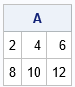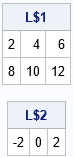SAS/IML programmers often create and call user-defined modules. Recall that a module is a user-defined subroutine or function. A function returns a value; a subroutine can change one or more of its input arguments. I have written a complete guide to understanding SAS/IML modules, which contains may tips for working with SAS/IML modules. Among the tips are two that can trip up programmers who are new to the SAS/IML language:

In the years since I wrote those articles, SAS/IML introduced lists, which are a popular way to pass many arguments to a user-defined module. Lists and matrices behave similarly when passed to a module:

• If you modify a list in a module, the list is also changed in the calling environment.
• If you specify an expression such as [A, B], SAS/IML creates a temporary list.

This article shows a subroutine that takes a list and modifies the items in the list. It also looks at what happens if you pass in a temporary list.

### Review: Passing a matrix to a module

Before discussing lists, let's review the rules for passing a matrix to a user-defined module. The following user-defined subroutine doubles the elements for its matrix argument:

```proc iml; /* Double the values in X. The matrix X is changed in the calling environment. */ start Double(X); X = 2*X; finish;   r1 = 1:3; r2 = 4:6; A = r1 // r2; /* each row of A is a copy. r1 and r2 do not share memory with A. */ run Double(A); print A;```As shown by the output, the elements of A are changed after passing A to the Double module. Notice also that although A was constructed from vectors r1 and r2, those vectors are not changed. They were used to initialize the values of A, but they do not share any memory with A.

Now suppose you want to double ONLY the first row of A. The following attempt does NOT double the first row:

```A = r1 // r2; /* each row of A is a copy */ run Double( A[1,] ); /* NOTE: create temporary vector, which is changed but vanishes */ print A;```

The result is not shown because the values of A are unchanged. The matrix is not changed because it was not sent into the module. Instead, a temporary matrix (A[1,]) was passed to the Double module. The values of the temporary matrix were changed, but that temporary matrix vanishes, so there is no way to access those modified values. (See the previous article for the correct way to double the first row.)

### Passing lists: The same rules apply

These same rules apply to lists, as I demonstrate in the next example. The following user-defined subroutine takes a list as an argument. It doubles every (numerical) item in the list. This modification affects the list in the calling environment.

```/* Double the values of every numerical matrix in a list, Lst. Lst will be changed in the calling environment. */ start DoubleListItems(Lst); if type(Lst)^='L' then stop "ERROR: Argument is not a list"; nItems = ListLen(Lst); do i = 1 to nItems; if type(Lst\$i)='N' then Lst\$i = 2*Lst\$i; /* the list is changed in the calling environment */ end; finish;   A = {1 2 3, 4 5 6}; B = -1:1; L = [A, B]; /* each item in L is a copy */ run DoubleListItems(L);/* the copies are changed */ print (L\$1)[label="L\$1"], (L\$2)[label="L\$2"];```As expected, the items in the list were modified by the DoubleListItems subroutine. The list is modified because we created a "permanent" variable (L) and passed it to the module. If you simply pass in the expression [A, B], then SAS/IML creates a temporary list. The module modifies the items in the temporary list, but you cannot access the modified values because the temporary list vanishes:

```/* create temporary list. Items in the list are changed but the list vanishes */ run DoubleListItems( [A, B] ); /* no way to access the modified values! */```

### Summary

This brief note is a reminder that SAS/IML creates temporary variables for expressions like X[1,] or [A, B]. In most cases, programmers do not need to think about this fact. However, it becomes important if you write a user-defined module that modifies one of its arguments. If you pass a temporary variable, the modifications are made to the temporary variable, which promptly vanishes after the call. To prevent unexpected surprises, always pass in a "permanent" variable to a module that modifies its arguments.

The post On passing a list to a SAS/IML module appeared first on The DO Loop.